Select Page

After successfully completing the CAT 2016 sprint series and the SNAP 2016 sprint series, we are back with the XAT 2017 sprint preparation series – Quant 4 to boost your prep. This series will consist of 10 sets of questions from past year XAT papers, leading to XAT 2017 and covered almost all the question types that you needed to know come the 8th of January.

# XAT 2017 sprint preparation series – Quant 4

Question Number 1 is followed by two statements labelled as I and II. Decide if these statements are sufficient to conclusively answer the question. Choose the appropriate answer from the options given below:

A.Statement I alone is sufficient to answer the question
B.Statement II alone is sufficient to answer the question
C.Statement I and Statement II together are sufficient, but neither of the two alone is sufficient to answer the question
D.Either Statement I or Statement II alone is sufficient to answer the question
E.Neither Statement I nor Statement II is necessary to answer the question

1. Given below is an equation where the letters represent (PQ).(RQ) = XXX. Determine the sum of P + Q + R + X.

1.X = 9.
2.The digits are unique.

Answer question numbers 2 to 4 based on the following information

Mulchand Textiles produces a single product of only one quality – waterproof synthetic fabric. Mr.Sharma, the cost accountant of Mulchand Textiles, estimated the costs of Mulchand Textiles for different possible monthly output levels. Before he could tabulate his estimates his computer crashed, and Mr. Sharma lost all data. Fortunately he had some printouts of some incomplete tables, charts and diagrams. The table titled “Variable Cost Estimates of Mulchand Textiles” provided the estimates of labour and material costs.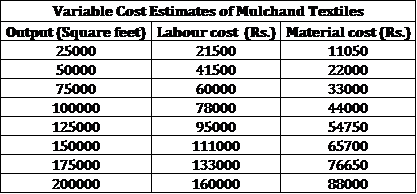Apart from labour and material costs Mulchand Textiles incurs administrative costs of Rs. 40,000 per month, and electricity costs. Mr. Sharma recalled that estimate data of variable electricity cost had certain peculiar characteristics. Values at every 25000 sq ft of output increased in geometric progression till 150000 sq ft of output, after which values increased in arithmetic progression for every 25000 sq ft of output. Mr. Sharma remembered that the electricity cost was estimated to be Rs. 3800 for 25000 sq. ft. of output, Rs. 5700 for 50000 square feet of output and Rs. 38856.50 for 175000 square feet of output.

2. The estimated cost per square feet of output is least for:

A. 75000 square feet output
B. 100000 square feet output
C. 125000 square feet output
D. 150000 square feet output
E. 175000 square feet output

3. The estimated material cost given in the table titled “Variable Cost Estimates of Mulchand Textiles” included the cost of material that gets spoiled in the production proces Mr. Sharma decomposed the estimated material cost into material spoilage cost and material usage cost, but he lost the data when his computer crashed. When he saw the following line diagram, he recalled that he measured the estimate of material spoilage cost per square feet of output on the y-axis and monthly output on the x-axis.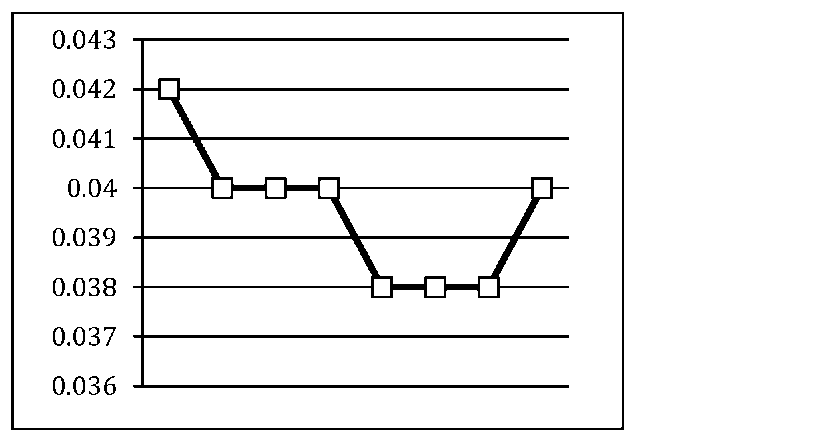Estimated material usage cost per square feet of output

A. Decreases up to 125000 square feet of monthly output and then increases monotonically
B. Recreases up to 50000 square feet of monthly output, remains constant between 50000 and l00000, decreases between 100000 and 125000, remains constant between 125000 and 175000 and finally increases between 175000 and 200000 of monthly output
C. Remains constant for all levels of monthly output
D. Increases up to 50000 square feet of monthly output, remains constant between 50000 and l00000, increases between 100000 and 125000, remain constant between 125000 and 175000 and finally decreases between 175000 and 200000 of monthly output
E. Increases up to 100000 square feet of monthly output and then decreases monotonically

4. Sharma found some printouts of line diagrams. The axes of the graphs were not marked, but Mr. Sharma remembered that he measured monthly output on the x-axis. Which of the following diagrams would represent the estimates of electricity cost per square feet of output versus monthly output?

A.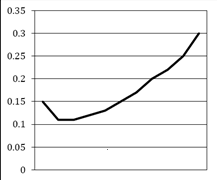B.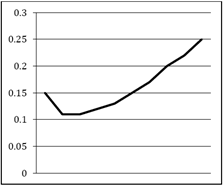C.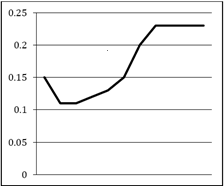D.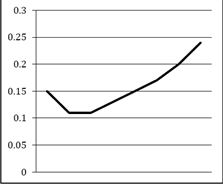E.5. Bee noticed something peculiar while entering the quiz marks of his five students into a spreadsheet. The spreadsheet was programmed to calculate the average after each score was entered. Prof. Bee entered the marks in a random order and noticed that after each mark was entered, the average was always an integer. In ascending order, the marks of the students were 71, 76, 80, 82 and 91. What were the fourth and fifth marks that Prof. Bee entered?

A. 71 and 82
B. 71 and 76
C. 71 and 80
D. 76 and 80
E. 91 and 80

6. Rakhal is looking for a field where he can graze his He finds a local farmer, Gopal, who agrees to rent his field to Rakhal for Rs. 1000 a year. Rakhal finds a post in the field and ties his cow to the post with a 25 feet rope. After some months, Gopal tells Rakhal that he will build a shed with four walls on the field with the post as one of the corner posts. The shed would be 15 feet by 10 feet. Rakhal agrees but he realizes that this arrangement would reduce the available area for grazing. What should be the modified rent to compensate for this loss of grazing area if Rakhal has to keep the cow tied to the same post with the same rope?

A. Rs. 800
B. Rs. 880
C. Rs. 888
D. Rs. 930
E. None of the above

7.There are two windows on the wall of a building that need repairs. A ladder 30 m long is placed against a wall such that it just reaches the first window which is 26 m high. The foot of the ladder is at point A. After the first window is fixed, the foot of the ladder is pushed backwards to point B so that the ladder can reach the second window. The angle made by the ladder with the ground is reduced by half, as a result of pushing the ladder. The distance between points A and B is

A. < 9 m
B. ≥ 9 m and < 9.5 m
C. ≥ 9.5 m and < 10 m
D. ≥ 10 m and < 10.5 m
E . ≥ 10.5 m

8. Amitabh picks a random integer between 1 and 999, doubles it and gives the result to Sashi. Each time Sashi gets a number from Amitabh, he adds 50 to the number, and gives the result back to Amitabh, who doubles the number again. The first person, whose result is more than 1000, loses the game. Let ‘x’ be the smallest initial number that results in a win for Amitabh. The sum of the digits of ‘x’ is:

A. 3
B. 5
C. 7
D. 9
E. None of these

9. Consider four natural numbers: x, y, x + y, and x – y. Two statements are provided below:

1. All four numbers are prime numbers
2. The arithmetic mean of the numbers is greater than 4

Which of the following statements would be sufficient to determine the sum of the four numbers?

A. Statement I.
B. Statement II
C. Statement I and Statement II
D. Neither Statement I nor Statement II
E. Either Statement I or Statement II

10. Triangle ABC is a right angled triangle D and E are mid points of AB and BC respectively. Read the following statements.

1. AE =19
2. CD = 22
3. Angle B is a right angle

Which of the following statements would be sufficient to determine the length of AC?

A. Statement I and Statement 2.
B. Statement I and Statement 3
C. Statement 2 and 3.
D. Statement 3 alone.
E. All three statements.

Solutions

1. As the last digit of XXX would be the product of two numbers that are the same, we can say that XXX could be either of 111, 444, 555, 666, or 999.

111 = 37*3 which is not possible.

444 = 37*12 which is not in the form of PQ * RQ

555 = 37*15 which is not in the form of PQ * RQ

666 = 37*18 which is not in the form of PQ * RQ

999 = 37*27 which is in the form of PQ * RQ

So, we do not require any of the statements and so, option e is correct.

2.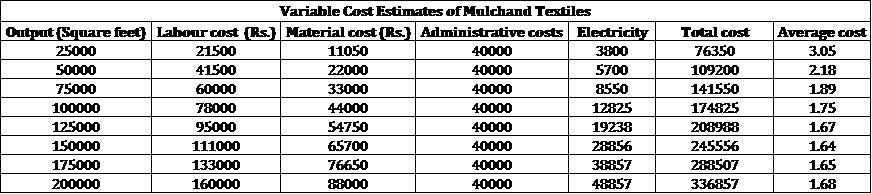Option d.

3.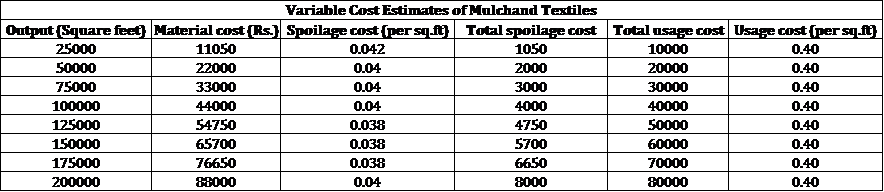All are the same. Option c.

4.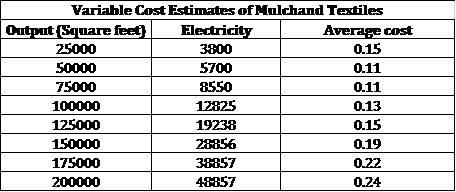Option b is correct.

5. The first two numbers have to be both even or both odd. Also, the third and the fourth numbers should be odd if the first two are even. So, either it is OOEEE or EEOOE. So, if the first two numbers are 71 and 91, the third number has to be a multiple of 3 which is not possible. So, the first two numbers have to be even. So, (76, 80) or (76, 82) or (80, 82). Only the second case is possible and so, the third number has to be 91. So, option c is correct.

6. Original area is 22/7*625.

New area will be ¾*22/7*625 + ¼*22/7*100 + ¼*22/7*225 = 22/7*550.

If original corresponds to 1000, new will correspond to 880. Option b.

7. Earlier distance is sqrt (30^2 – 26^2) = approximately 15. cos 2x = 15/30 and so, x = 30 degrees. So, new distance from the base will be 15√3 and so, the difference will be 15*0.732 = 10.980. Option e.

8. Amitabh picks x, gives Sashi 2x, Sashi gives 2x + 50, Amitabh gives 4x + 100 and so on. Amitabh has to win and so, the number with Sashi could be 2x + 50, 4x + 150, 8x + 350, 16x + 750. So, as 16x + 750 > 1000, minimum value of x will be 16. Option c.

9. If statement I is true, one of the base numbers has to be 2. x, 2, x + 2 and x – 2 are prime. By observation, x = 5 is possible. Post that, we do not have three consecutive odd numbers that are prime. So, statement I is sufficient.

If statement II is true, 3x + y > 16. This could give us multiple cases and so, this statement is not sufficient.

Option a.

10. Using all three statements only can we find out the length of AC. Option e.

What’s hot at Learningroots?

You can follow the entire sprint series here: XAT 2017 Sprint Preparation Series by Learningroots

error: Content is protected !!